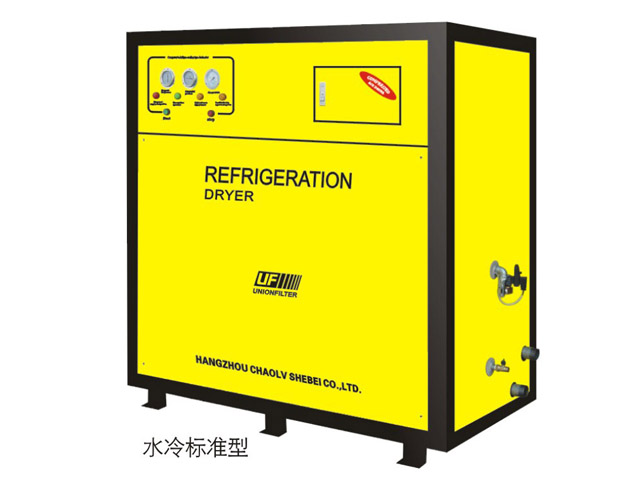# 如何计算冷干机的热负荷？

In a cold dryer, the load of the heat exchanger (evaporator and precooler) can be calculated by calculating the enthalpy difference between the inlet and outlet of the thermal fluid.

Δh=1.01Δt+（2501+1.84Δt）Δd （KJ/kg干空气） ①
Delta h=1.01 Delta t+ (2501+1.84 delta T) Delta D (KJ/kg dry air)

In the form of delta T - the temperature difference between the inlet and outlet of the compressed air as a thermal fluid, C

Δt=t’-t”
Delta t=t '-tt’──压缩空气进入换热器时的温度
T '-- the temperature of compressed air entering the heat exchanger

t”──压缩空气离开换热器时的温度
T "- the temperature of compressed air leaving the heat exchanger

1.01──干空气的平均定压比热，KJ/kg.℃
1.01 - average constant pressure and specific heat of dry air, KJ/kg. C

1.84──水蒸气的平均定压比热，KJ/kg.℃
1.84 - the average constant pressure of water vapor, KJ/kg. C

2501──水的汽化潜热，KJ/kg
2501 - latent heat of vaporization of water, KJ/kg

Δd ──换热器中产生的凝结水量，kg
Delta D - condensation water produced in the heat exchanger, kg

Δd=d’-d”
Delta d=d '-d

In the formula, d 'and D respectively indicate the saturated water content in the compressed air, kg, when the temperature T' and T are corresponding to the temperature.

The heat load of the heat exchanger is

Q=qmΔh （W） ②
Q=qm delta H (W) 2

In the formula, QM - the mass flow of air, kg/s

The formula 1 and 2 are the basic formulas for calculating the heat load of the compressed air cooling process. It consists of the following three parts:

1）1kg干空气从t’降到t”放出的热量1.01Δt；
1) the heat of 1kg dry air from t 'to t' is 1.01 delta T;

2）（d’-d”）kg的水蒸气从t’降到t”放出的热量；
2) the heat released from the water vapor from t 'to t in (d' -d ") kg.

3）（d’-d”）kg的水蒸气凝结成液态水放出的凝聚热（在数值上等于汽化潜热）。
3) (d '-d ") the condensation heat of the water vapour of kg condenses into liquid water (in numerical value equals vaporization latent heat).

Among them, 1), 2) two is the heat that changes with the temperature. It is called sensible heat. Third) is determined by the amount of condensation water. It does not participate in the heat exchange of the system, so it is latent heat.

In evaporator, the cooling capacity (or absorbed heat) released by the refrigerant evaporation process is used for cooling the compressed air, and the other part is used to cover the latent heat required for steam condensation.

In the pre cooler, the amount of heat absorbed (or released) during the heating process of low temperature and dry air is partly used for cooling the compressed air intake, and the other part is used to cover the latent heat required for condensation of some steam in the hot gas flow.

Combined with the above picture, the heat load of the precooler can be written when the unit mass flow (kg/s) is compressed into the air.

Q’=[1.01Δt1-2+（2501+1.84Δt1-2）*（d1-d2）] （W）
Q '=[1.01 Delta t1-2+ (2501+1.84 delta T1-2) * (d1-d2)] (W)

In the formula, Delta t1-2=t1-t2

When the air is compressed by a unit mass flow (kg/s), the heat load of the evaporator can be written as the following expression

Q0=[1.01Δt2-3+（2501+1.84Δt2-3）*（d2-d3）] （W）
Q0=[1.01 Delta t2-3+ (2501+1.84 Delta T2-3) * (d2-d3)] (W)

In the formula, Delta t2-3=t2-t3